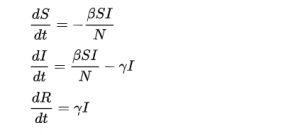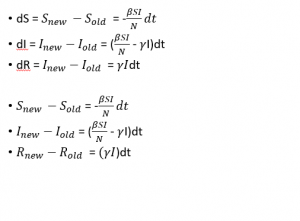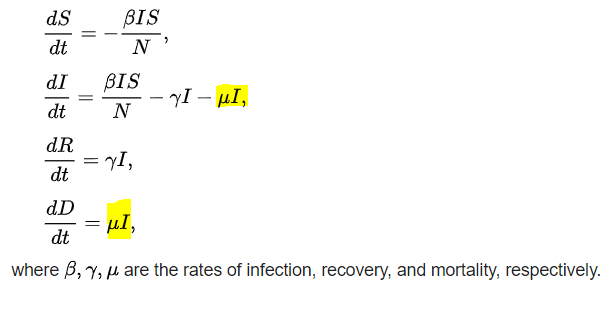Idowu Ilekura — August 10, 2021

This article was published as a part of the Data Science Blogathon

## Introduction

According to Haines and Crounch, mathematical modelling is a process in which real-life situations and relations in these situations are expressed by using mathematics. In simpler terminologies, mathematical modelling is the process of describing systems (activities) with mathematics. Mathematical modelling is the process of using mathematics to model real-world processes and occurrences.

Mathematical modelling is used virtually in every sector, in the manufacturing industry mathematical modelling is used to model heat and mass transfer of fluids flow, the transformation of materials, e.t.c. The construction industry is not spared from the beauty of mathematical modelling, mathematical modelling is used to optimize the amount of port in structures, calculating the stress that will be imposed on buildings and how to counterbalance it. You probably must have seen the tallest building in the world either virtually or physically, you will be in awe if you were to see all the mathematical models that were used to model the building.

.

Football athletes use mathematical modelling to score goals, for football lovers you probably must have seen how Messi, Rolando, and other popular footballers use free kicks to score goals. The free-kick goals can be modelled with mathematics, by modelling the angle of trajectory, the drag e.t.c.

The astronomy industry heavily relies on mathematical modelling, mathematics is used to model the movement of spacecraft and other orbital objects. Katherine Johnson, a former mathematician at NASA used her mathematical prowess to help put an astronaut into orbit around the earth. Her mathematical skills were also used to deploy a man on the moon.

I can continue to list the sacrosanct role of mathematics in our world, but because of time constraints, I will stop here. The reality is that the world can exist without the English Language but the world can’t exist without Mathematics.

This article will walk you through the processes of modelling disease spread with mathematical models. You might be wondering can mathematics really model disease spread? The answer is yes, mathematics is very important in the health sector. According to TheConversation “Mathematical models are used to create a simplified representation of infection spread in a population and to understand how an infection may progress in the future. These predictions can help us effectively use public health resources such as hospital space or a vaccination programme. For example, knowing how many people in a population are likely to become infected can tell hospitals how much space and resources they will need to allocate for treatment.”

What it takes to mathematically model any disease

At the beginning of an epidemic, there exist, people who will be infected, prone to getting infected and those who might recover from the disease or die as a result of the disease. Those who were initially prone to the disease will get infected if they come in contact with infected people and those who will die will originate from the infected people. Mathematicians have been trying to successfully find a way to mathematically model the relationship between those who are prone to be infected, those who are infected and those who will recover from the disease. In 1927, Kermack & McKendrick came up with what is called the Susceptible, Infected and Recovered (SIR) Mathematical model. The SIR model assumes that for any given disease, there exist 3 categories of people those who are Susceptible (Prone to contracting the disease but are yet to be infected), those who are Infected and those who have been Removed(recovered) (either by death or with the aid of drugs). The SIR model has been of help to mathematicians and has made modelling disease spread easy.

SIR Image
To mathematically model any disease using the SIR model, you will need to assume that the population remains constant i.e ( No birth takes place, nobody migrates into the population, no natural death ( with an exception of death from the disease)). The SIR model models diseases by taking into cognizance that, the movement of people from the Susceptible into the Infected state and from the Infected State into the Removed state is defined by some constants. These constants are the tripod that the SIR model sits on, and that is what will be discussed soonest. You will agree with me that, for any disease to spread there must be contact between susceptible people and infected people or person( disease carriers).

Assuming for a particular epidemic, there exist 1000 Susceptible people and 3 persons that are infected. Take, for instance, every day 1 person gets infected due to the contact between Susceptible and Infected people. You will agree with me that, on the fifth day, 8 people will be infected and the number of susceptible will be 995. We might want to assume that 2 persons or 3 persons get infected, one thing here is that we are just making assumptions that might not be mathematically accurate. Hence the need to use the SIR model to mathematically and accurately model the spread of the disease.

The SIR model models the number of people who are infected by assuming that everyone in the susceptible category has an equal probability of being infected by a constant fraction which is called the contact rate (infection rate). The number of people that are infected is computed by multiplying the contact rate with the number of infected people and the Susceptible after which the population number is used to divide the result i.e (contact rate * S * I)/N. S-Susceptible, I– Infected, and N– Total Population Number.  The contact rate will be a fraction of the population which is computed by analyzing the number of contacts made with infected people per day. The SIR model also models the number of people who will be removed by a certain fraction which is called the recovery rate. The number of people that will be removed is computed by multiplying the recovery rate with the number of infected people i.e recovery rate * infected people.SIR Mathematical Model

• ds/dt = the rate of change of the susceptible over time
• dI/dt is the rate of change of infected over time
• dR/dt is the rate of change of removed over time

The equation simply states that susceptible people will be reduced over time based on the contact rate (beta), the number of susceptible, the number of infected, and the total population (N). You will notice the presence of the negative sign, this is to show the fraction of people that will be lost from the susceptible category. The fraction of people that are lost from the susceptible category will be added to the infected category, hence the presence of the positive sign in the infected equation. Recall that the removed people originate from the infected category and the number of people that are removed is based on the removal rate multiplied by the number of infected people (gamma * I). Those that are removed will be a loss to the infected people hence the need to subtract the number of removed from the number of infected. The removed people will be gain to the removed category, hence the positive sign for the removed category.

Multiplying both sides with dt will give

dS is the rate of change i.e the difference between the old susceptible and the new susceptible ( Snew– Sold). The number of susceptible, infected, and Recovered for the next day can be modeled by moving the old Susceptible, Infected, and Recovered numbers to the other side of the equation to give.The above equation can be used to model the number of susceptible, infected, and recovered for the next day. The number of infected people in a day depends on the contact rate(Beta) and the recovery rate (gamma).

## Other Types of Mathematical Models Used to Model Diseases

SIID or SIRD Model
Apart from the SIR model, several varieties of mathematical models can be used to model diseases. Other models that were derived from the SIR models are the SEIR model, SIRV model, SIRD model e.t.c. The SEIR model models disease based on four-category which are the Susceptible, Exposed (Susceptible people that are exposed to infected people), Infected, and Recovered(Removed). The Susceptible, Infected, Recovered(Removed) and Vaccinated(SIRV) is another type of mathematical model that can be used to model diseases. The focus of this article is on the SIRD or SIID model which is Susceptible, Infected, Removed(Recovered with immunity), and Dead or Susceptible, Infected, Immune, and Dead model.

The SIID or SIRD model is an extension of the SIR model with an addition of two assumptions which are recovery with immunity and Death. For the rest of this article, I will interchange SIRD for SIID, both refer to the same acronym.SIRD image showing the mortality rate. Image by Author.muI that is coloured with yellow is the mortality rate.

## Simulating Diseases with SIRD(SIID) (Practical)

The disease that we will be modelling is called Konvid-18 disease, let’s assume Konvid-18 has been detected in JavaGo city with a total population of 1000, our aim is to model the disease to better advise the authorities and the people of JavaGo city. Konvid-18 is a deadly disease that kills, those who contract Konvid-18 can recover and be immune or die.

Given the above information that immunity exists and people die as a result of the disease, it means we will use the SIID model to model the disease. Let’s assume the number of people who are infected by the disease is 3, the number of dead and recovered is zero, the infection rate(beta) is 0.5, the recovery rate(gamma) is 0.035 and the death rate(mu) is 0.005. Note that the infection rate, recovery rate, and death rate were gotten from , but you can try any number.

The Susceptible number for the next day can be computed by using this method

Snew = Sold – (beta * Sold* Iold )/N

Sold = N – Iold = 1000-3 = 997 (i.e the susceptible number for the current day is the difference between the total population and the number of infected people in the current day)

beta = 0.5

Iold  = 3

N = 1000 (The total Population)

Snew = 997 – (0.5 * 997 *3 )/1000

Snew = 997 – 1.4955

Snew = 995.5045

The total number of Susceptible for the next day is approximately 995.5

Let us compute the rest, the next day number of infected can be computed with this method

Inew = Iold + (((beta * Sold * Iold)/N) – (gamma * Iold) – (mu * Iold))

gamma ( recovery rate) = 0.035

mu (death rate) = 0.005

Inew = 3 + ((0.5 * 997 * 3)/1000) – (0.035 * 3) – (0.005 * 3))

Inew = 3 + (1.4955 – 0.105 – 0.015)

Inew = 3 + 1.3755

Inew = 4.3755

The number of people that will be infected the next day is approximately 4.4

Modeling the number of people that would have recovered with immunity the next day, that can be modelled with this equation.

Rnew = Rold + gamma * Iold

Rold = 0

Rnew = 0 + 0.035 * 3

Rnew = 0 + 0.105

Rnew = 0.105

The number of people who would have recovered with immunity the next day is approximately 0.11

Lastly, modelling the number of people who would be dead the next day, this method can be used which is the application of the last equation

Dnew = Dold + mu * Iold

Dold = 0 + 0.005 *3

Dold = 0 + 0.015

Dold = 0.015

These steps can be repeated to model the number of susceptible, infected, recovered and dead for the next 2 days and more days. What if the steps can be automated, instead of manually computing the numbers. Python Programming language will be used to automate the process and plot the result.

## Modelling Disease with Python Programming

#### Prerequisites

To follow along, you will need to have python and preferably Jupyter notebook installed on your system. You can use this link to download anaconda, anaconda comes with a Jupyter notebook and python. You can use this video to familiarize yourself with the Jupyter notebook and how to install it.

Now that you have Jupyter notebook installed, you are good to go. Let us fire down

```# importing neccessary libraries

import matplotlib.pyplot as plt

%matplotlib inline

# defining the variables

total_population = 1000

total_infected = 3

total_susceptible = total_population - total_infected

total_recovered = 0

# Number of days to simulate disease

simulation_days = 500

# list to store the numbers of recovered people with immunity over time

# the first element will be the initial number of people that has recovered with immunity

recovered_list = [total_recovered]

#list to store the number of dead people over time

infected_list = [total_infected]

susceptible_list = [total_population]

infection_rate = 0.5

recovery_rate = 0.035

death_rate = 0.005

#using the range function to simulate for 500 days which is the simulation days

for days in range(1,simulation_days):

num_infected_daily = (infection_rate * total_infected * susceptible)/total_population
# get the susceptible number for next day
total_susceptible = total_susceptible - num_infected_daily
num_recovered_daily = recovery_rate * total_infected

total_infected = total_infected + (num_infected_daily - num_recovered_daily - num_dead_daily)
total_recovered = total_recovered + num_recovered_daily

susceptible_list.append(total_susceptible) # adding to the list of susceptible people
infected_list.append(total_infected)
recovered_list.append(total_infected)

Now that we have simulated Konvid-18 for 500 days, we can now visualize our result.

Visualizing the result

```# Using plt.plot to plot
plt.plot(range(0,simulation_days),susceptible_list,color='blue',label='Susceptible')
plt.plot(range(0,simulation_days),infected_list,color='red',label='Infected')
plt.plot(range(0,simulation_days),recovered_list,color='green',label='Recovered)
plt.legend() #add the labels to the plot
plt.title('Konvid-18 Disease Simulation in JavaGo city')
plt.xlabel('Days')
plt.ylabel('Total Population')
plt.show()```

After running the above code, the image below will be displayed.

## Deductions

From the above plot, it can be seen that on day 100, the numbers of susceptible people have dropped drastically to below 200, while the number of recovered was above 200 and the number of dead people is less than 100. The number of infected people was at its peak on day 100 after which there was a steady decline. You can add any insights that you were able to derive from the plot in the comment box section.

## Conclusion

The article has shown you the importance of mathematical models, how to model diseases with the SIRD model, how to automate the process for days, and how to visualize it. The article introduced you to the SIRD model, there are other mathematical models that you can explore further and dive deeper into like the SEIR, SIS, SIRV e.t.c. The article also didn’t cover the mathematics of deriving the contact ratio, recovery rate, and death rate, you can explore these concepts further. I hope you have realized the importance of mathematics in the healthcare industry.

I created a demo web app for further exploration, the web app was developed with streamlit. You can access the web app with this link and check the source code with this link.

You can connect with me on LinkedIn,

## References/More Resources

(1) https://en.wikipedia.org/wiki/Compartmental_models_in_epidemiology#The_SIR_model